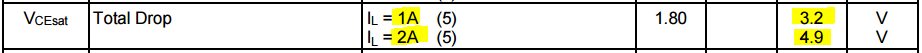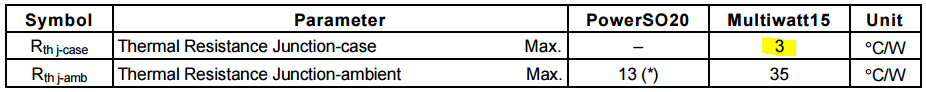Heat Sink Design Guide

In a world of ever-increasing electronics, our lives are continuously impacted by devices that incorporate some sort of heat-generating circuitry. When it comes to electronics that dissipate power in the form of heat during normal operation, it is often necessary to manage this heat, to ensure the components don’t exceed their maximum allowable temperatures.

One of the most common methods to manage power dissipation is through the use of a heat sink. Heat sinks are used on a broad range of electronics, ranging from CPUs to motor drivers.

In this article, we'll walk you through the basics of heat sinks and heat sink design, including the calculations involved in defining the proper heat sink for your application. In our example of proper heat sink design, we’ll use the common L298 (multiwatt15) full bridge driver, as this chip can get very hot when operating at the upper limit of its range.

What are Heat Sinks?

A heat sink is a mechanical component that is attached to an electrical component for the sake of transferring heat from the electrical component into the surrounding environment. This environment is most commonly air, but it can also be other fluids, such as water or coolant. Heat sinks are typically made of aluminum or copper and have fins or pins that work to increase the surface area of the component, to expedite the heat transfer to the surrounding fluid. The image below demonstrates both a fin (right) and a pin (left) type of heat sink.As we can see, the heat sink has a much greater surface area than the flat component to which it would be attached. The metals of which heat sinks are made also have a much higher coefficient of thermal conductivity than the plastic packaging of typical electrical components. This article will walk through the math behind heat sinks, using a real-world example. It may help the reader to review the data sheet for the L298 Dual Full-Bridge Driver to help with following along.

Establishing the need for a Heat Sink

We know what heat sinks do, but why do we actually need them? Is there really a problem with components getting hot? The answer is definitely YES. Circuits have a maximum operating temperature that is typically referred to as the maximum junction operating temperature (T op). The L298 specifies this as 130°C in the datasheet. We cannot exceed this temperature, or damage is likely to occur. We also know that the component will generate heat during standard operation and that it will operate in an environment with a given ambient temperature. That means we have to find a way to quantify the amount of heat that will be generated during normal operation, as well as how this will impact the operating temperature in our ambient surroundings. Luckily, we have formulas to help us get there. The formula below defines the maximum amount of power that we can dissipate, given a few known variables:Where:The ambient temperature is room temperature for open electronics that are indoors but may be higher for projects that are enclosed with other heat-generating devices. It will be even higher than that if a heat-generating device is located outside, in the hot summer sun.

The thermal resistance is a value that defines the amount of temperature rise per watt of power dissipation within the component. If we look in the datasheet for the L298, we can see that the thermal resistance from the junction to ambient is 35 C/W. That means that for each watt we dissipate, the chip will raise in temperature by 35 degrees. If we plug these values into the formula above, we get the following:However, we know the chip is rated to 2A per channel, with an input voltage of 46V. This puts us at 92 watts, right? While that is correct for the total power input, not all of it is lost to heat in the chip. This calculation will be covered below, but it is safe to say that the chip will need to dissipate more than 3.06W of heat to get anywhere near the maximum current rating.

Calculations to Size a Heat Sink, L298 Max Power

As mentioned above, we’re going to dive into this analysis with a real-world example of the L298. We’ll do this in a logical order, but it doesn’t always have to occur in this sequence, depending on the information you have. In our example, we’ll start by characterizing the component and then move into heat sink selection.

Power Dissipated in the IC

The power that is dissipated in the component won’t be the full operating load, but we can determine what it actually is by looking through the data sheet. In the image below, we can see that the L298 will have a voltage drop of 4.9 V at the max continuous current of 2A and a drop of 3.2V at 1A.This is equal to 9.8 watts and 3.2 watts, respectively. We can already see that the chip will overheat if running 1A in room temperature surroundings. This establishes the need for a heat sink, even at 1A continuous.

Thermal Resistance

Previously, we mentioned thermal resistance in one of our equations. Thermal resistance is a variable used to describe the relative resistance of heat transfer that is present in a component package. For the initial example, we used 35C/W as our thermal resistance because this is what the data sheet specifies for the component. However, there is more to thermal resistance than just this data sheet value. If you look at the data sheet excerpt below, we can see that there is also a value for thermal resistance from the junction to the case.This describes how heat is transferred from the junction to the case but neglects the heat transfer from the case to the ambient surroundings. That’s where the next variable (Rth j-amb) comes into play. However, if we use a heat sink and thermal compound, we can improve the heat transfer to the surrounding air. What we end up with is a system of thermal resistances that need to be lower than the total resistance of the original component to ambient (35 C/W). The image below (not an L298) illustrates the different sources of thermal resistance with a heat sink assembly.We can see that the heat will have to transfer through the case, thermal compound, or adhesive (indicated by the blue dashed line) and then through the heat sink to the air. It is important to note that I am using the term “thermal compound” to represent any thermal interface material, such as paste, grease adhesive, or pad. Since we have multiple resistances, our total thermal resistance of the system will be the sum of all three sources. That is to say that we need to use the equation below to determine our new thermal resistance:Now that we know how thermal resistance impacts our design, we need to calculate the required thermal resistance for our load case to determine which heat sink will work, or if one even exists.

Calculating the Required Thermal Resistance to Accommodate Power Dissipation

If you recall our first equation, we solved for max power dissipation. We can rearrange this equation to determine the required thermal resistance for a known power dissipation, as shown below.Using this equation, we can see that for 1A, we need 33.4 C/W, and for 2A, we will need an even better thermal resistance of 10.9 C/W. We also know that this resistance will be driven by a system of three components, so let’s see whether we can achieve this in the next section.

Selecting the Heat Sink and Compound

Of the three resistances, we can really only control the last two because the component’s thermal resistance is defined by the manufacturer. If we write it out as an equation, we know that the combined thermal resistance of the heat sink and the thermal compound are represented as shown below for both 1A and 2A applications:Now, we need to look at our options for both thermal compounds and heat sinks. The table below details a few thermal compounds and their respective resistance, based on information from Wakefield Thermal Solutions.We can see that the lower thermal resistance is given by the 122 Series compound, but they are all at or below 0.5 C/W, so we will pick this as a conservative value. Next, we can subtract this thermal resistance from our value in the equation above to derive the proper thermal resistance for a heat sink. This is shown numerically below:This leaves us with the need for a heat sink that has a thermal resistance of 29.9 C/W or less for 1A and 7.4 C/W or less for 2A. Now we can start searching our vendors to see if this heat sink is available or if we need to reduce our maximum operating load. Luckily, the AAVRID 6400 BG has a thermal resistance of just 2.7 C/W, which meets both of our criteria. Our total thermal resistance is now equal to:Final Calculation

Now that we have our heat sinks selected, let’s look at the equation to get an estimate as to the maximum temperature of the chip for our two load cases. We will rearrange our first equation to get the operating temperature with the new operating temperature, as shown below:While this is a great start, and it gives us confidence in the setup we have chosen for our thermal management solution, it’s important to test this configuration. This can be done by running the part at your max intended loading and monitoring the temperature, to determine if it is in line with what you expect.

Additional Factors that Impact Heat Sink Performance

Maybe you can’t get the temperatures as low as you wanted for the component, even if you thought your heat sink design was perfect. There are other ways of bringing the temperature down that work in conjunction with the heat sink. Some of the key variables are:

• Thermal Compound Conditions – Not only do we want a well rated thermal compound, we also want to ensure it is only as thick as it needs to be to fill the gap between the component and heat sink. If the gap is too thick, it may slow heat transfer.

• Natural and Forced Convection – Since a heat sink works by dispersing heat to the surrounding air, the process can be improved by moving that air parallel to the fins of the heat sink. This will lower the effective thermal resistance of the heat sink, while ensuring that the ambient air remains at a lower temperature. On that same note, if the heat sink is packaged in a very small package with restricted air flow, natural convection is hampered and may reduce the effectiveness of the heat sink.

These factors are another reason to test your configuration before large-scale use or production.

Main Takeaways

Selecting the proper heat sink is an important factor in ensuring the reliable operation of your design. The formulas and examples in this article should put you on your way to improving the thermal management of your product. If you want to push the limits of thermal management, I encourage you to take the next step and read more about heat transfer and fluid dynamics.